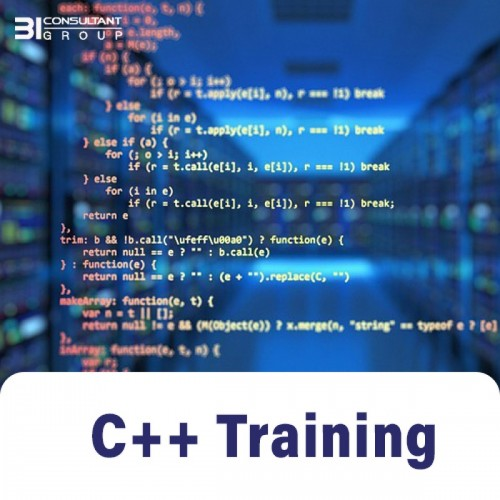# C++ Training### C++ Training

C++ is a cross-platform, object-oriented programming language that developers use to code high-performance operating systems, graphical user interfaces, and applications. C++ can also be used as an extension of, or in collaboration with, C#, Java, Python, and various other programming languages. Programmers may find learning C++ helpful because it’s a portable language with a clear structure and reusable code.Those who learn C++ may find that the language provides a high level of flexibility and control over system memory and resources. C++ programming is also highly adaptable with other programming languages, which helps programmers create different application versions for various platforms.

#### Price: \$750.00

The training course is designed to meet the needs of modern learners, we provide hands-on experience and this course can help you master C++ basics that can come in handy throughout your career. C++ training program with BI Consultant Group can teach C++ syntax, C++ functions, C++ programming fundamentals, and more.

Moreover, advanced C++ course modules provide in-depth lessons on the programming language, which can include hands-on experience creating applications on various platforms and operating systems, including Windows, Linux, UNIX, and macOS. Courses may cover memory management systems, advanced C++ functions, mechanics, templates, and more.

Prerequisites

• Anybody with an interest in learning a programming language that will open the doors for further career progression
• Knows the basic functionality of computer programs

Target Audience

• Beginners who want to learn computer programming from Basic to Advanced level
• Engineering Students and Working Professionals who want to get hands-on experience of computer programming

Outcomes

After the completion of the program, learners can apply for job roles in:

• Software developer
• Operating system developer
• Application programmer
• Computer graphics programmer
• Database programmer
• Web developer
• Network programmer
• Electrical/ Mechanical/ Mechatronics Industry
• Manager Operations
• Industrial roles

Duration:

40+ hrs

## Course Contents

• FLOWCHARTS

Introduction to flowcharts, Decision making using flowcharts, Loops, Example problems

• VARIABLES AND DATA TYPES

First program, Variables and data types, Taking input, How data is stored in memory, Arithmetic Operators

• CONDITIONAL STATEMENTS

Introduction to If else, Relational and logical operators, Nested conditionals

• WHILE LOOPS

While loops, Flow of execution of statements in while loop, Example problems using while loop

• PATTERNS

Introduction to patterns, Basic Patterns, Square Patterns, Triangular Patterns, Character Patterns, Reverse Triangle, Inverted patterns, Isosceles triangles

• FOR LOOPS

For loops, Break and Continue, increment - decrement operators

• FUNCTIONS

Introduction to functions, Working of function calling, Variables and its scope, Pass by value

• INTRODUCTION TO ARRAYS

Introduction to arrays, How arrays are stored in memory, Passing arrays to functions

• SEARCHING AND SORTING

Understanding Binary Search, Selection sort, Bubble sort, Insertion sort, Merging two sorted arrays

• STRINGS

Introduction to strings, storage of strings and their inbuilt functions

• 2D ARRAYS

2D arrays, Storage of 2D arrays, Example problems using 2D Arrays

• RECURSION
• Introduction to recursion, Principle of mathematical induction, Fibonacci numbers, Recursion using arrays, Recursion using strings, Recursion using 2D arrays
• TIME AND SPACE COMPLEXITY

Order complexity analysis, Theoretical complexity analysis, Time complexity analysis of searching and recursive algorithms, Theoretical space complexity, Space complexity analysis of merge sort

• BASICS OF OOPS
• Introduction to OOPS, Creating objects, Getters and setters, Constructors and related concepts, Inbuilt constructor and destructor, Example classes
• Static members, Function overloading and related concepts, Abstraction, Encapsulation, Inheritance, Polymorphism, Virtual functions, Abstract classes, Exception handling
• STACKS AND QUEUES
• Introduction to stacks, Stack using arrays, Dynamic Stack class,Stack using linked list, Inbuilt stack, Queue using arrays, Dynamic queue class, Queue using linked list, Inbuilt queue
• GENERIC TREES
• Introduction to Trees, Making a tree node class, Taking a tree as input and printing, Tree traversals, Destructor for tree node class
• BINARY TREES
• Introduction to Binary Trees, Taking a binary tree as input and printing, Binary Tree traversals, Diameter of binary tree
• BINARY SEARCH TREES
• Introduction to Binary Search Trees, Searching a node in BST, BST class, Inserting and Deleting nodes in BST, Types of balanced BSTs
• PRIORITY QUEUES
• Introduction to Priority Queues, Ways to implement priority queues, Introduction to heaps, Introduction to Complete Binary Trees and its implementation, Insert and Delete operations in heaps, Implementing priority queues, Heap sort, Inbuilt Priority Queue
• HASHMAPS
• Introduction to Hashmaps, Inbuilt Hashmap, Hash functions, Collision handling, Insert and Delete operation implementation in hashmap, Load factor, Rehashing
• TRIES
• Introduction to Tries, Making a Trie Node class, Insert, Search and Remove operation implementation in Tries, Types of Tries, Huffman Coding
• GRAPHS
• Introduction to Graphs, Graph Terminology, Graph implementation, Graph Traversals (DFS and BFS), Weighted and Directed Graphs, Minimum Spanning Trees, Cycle Detection in Graphs, Kruskal's algorithm, Prim's Algorithm, Dijkstra's algorithm
• INTRODUCTION TO DYNAMIC PROGRAMMING
• Introduction to Memoization, Introduction to Dynamic Programming, Fibonacci numbers using recursion, memoization and dynamic programming
• APPLICATIONS OF DYNAMIC PROGRAMMING

Longest Common Subsequence (LCS) using recursion, memoization and dynamic programming, Edit distance using recursion, memoization and dynamic programming, Knapsack problem using recursion, memoization and dynamic programming